# Integrated Applications in Entris®️ II

Whether you need to perform common weighing procedures or run dedicated laboratory applications, Entris®️ II will give you the best of both. It provides 12 built-in applications as standard features. They provide built-in applications with GLP | GMP Compliant Printout and Data Output.### Animal Weighing

Averages multiple individual measurements for accurate weighing of moving samples (for instance live animals) and for weighing in unstable environments. The weighing process can be started manually or automatically.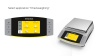### Check Weighing

Checks if a sample weight is within the specified tolerances, with easy visualization through clear messages in the display. The weighing results can be printed automatically as defined in setup of the application.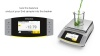### Components

Enables you to weigh individual components into different containers and then retrieve the total amount weighed-in.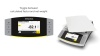### Conversion

The balance displays the weight in the selected weighing unit. In addition you can toggle between 5 different user-defined weighing units.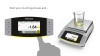### Counting

Determines the number of identical parts with reference to a known defined weight. The balance will show you the calculated quantity and the total weight.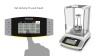### Density Determination

Determines the density of solids using the buoyancy method. Weigh the sample first in air and then submerge in liquid, and the application calculates the sample density automatically.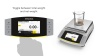### Mixing

Records successive weight values of different components of a recipe as they are added to a vessel, with taring between each component. Records and documents the values of all individual components, as well as the total weight value.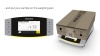### Peakhold

Captures and stores the highest positive stable weight value (peak), so that you can read it off for 5 sec after removal of the object.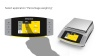### Percentage Weighing

Determine the percentage share of an unknown sample in comparison to a reference weight. Weigh the reference weight first, which defines the percentage, then weigh your unknown sample and the application calculates the percentage of this sample compared to the reference.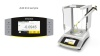### Pipette Smart Check

Check the repeatability of your pipette on a smart way by dosing a certain number of the same volume. Statistically analyzes the repeatability by: Number of measurements (dosing cycles), Average mean weight, Standard deviation, Variation coefficient, Sum of all weights, Lowest weight value (min.), Highest weight value (max.), Difference between min. and max.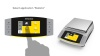### Statistic

Saves a certain number of weight values (components) and statistically analyzes them. Statistical analyses include: Number of components, Average mean weight, Standard deviation, Variation coefficient, Sum of all weights, Lowest weight value (min.), Highest weight value (max.), Difference between min. and max.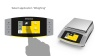### Weighing

Measures the weight of a sample with high accuracy and includes the ability to assign individual sample and lot IDs. Weighing results can be printed and exported manually or automatically.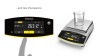### Weighing | Dosing

Measures the weight of a sample with high accuracy and includes the ability to assign individual sample and lot IDs. Weighing results can be printed and exported manually or automatically.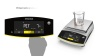### Counting

Determines the number of identical parts with reference to a known defined weight. The balance will show you the calculated quantity and the total weight.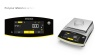### Percentage Weighing

Determine the percentage share of an unknown sample in comparison to a reference weight. Weigh the reference weight first, which defines the percentage, then weigh your unknown sample and the application calculates the percentage of this sample compared to the reference.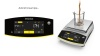### Mixing | Net Total

Records successive weight values of different components of a recipe as they are added to a vessel, with taring between each component. Records and documents the values of all individual components, as well as the total weight value.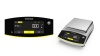### Components | Totalizing

Enables you to weigh individual components into different containers and then retrieve the total amount weighed-in.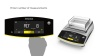### Animal Weighing

Averages multiple individual measurements for accurate weighing of moving samples (for instance live animals) and for weighing in unstable environments. The weighing process can be started manually or automatically.### Calculation | Free Factor

Converts a unit of mass using a defined factor. For instance, the application can calculate the weight per unit area, such as gsm. This application will show you the converted weight at the touch of a key.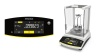### Density Determination

Determines the density of solids using the buoyancy method. Weigh the sample first in air and then submerge in liquid, and the application calculates the sample density automatically.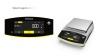### Statistics

Saves a certain number of weight values (components) and statistically analyzes them. Statistical analyses include: Number of components, Average mean weight, Standard deviation, Variation coefficient, Sum of all weights, Lowest weight value (min.), Highest weight value (max.), Difference between min. and max.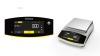### Peak Hold

Captures and stores the highest positive stable weight value (peak), so that you can read it off for 5 sec after removal of the object.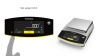### Check Weighing

Checks if a sample weight is within the specified tolerances, with easy visualization through clear messages in the display. The weighing results can be printed automatically as defined in setup of the application.

### Mass Unit Conversion

The balance displays the weight in the selected weighing unit. In addition you can toggle between 5 different user-defined weighing units.